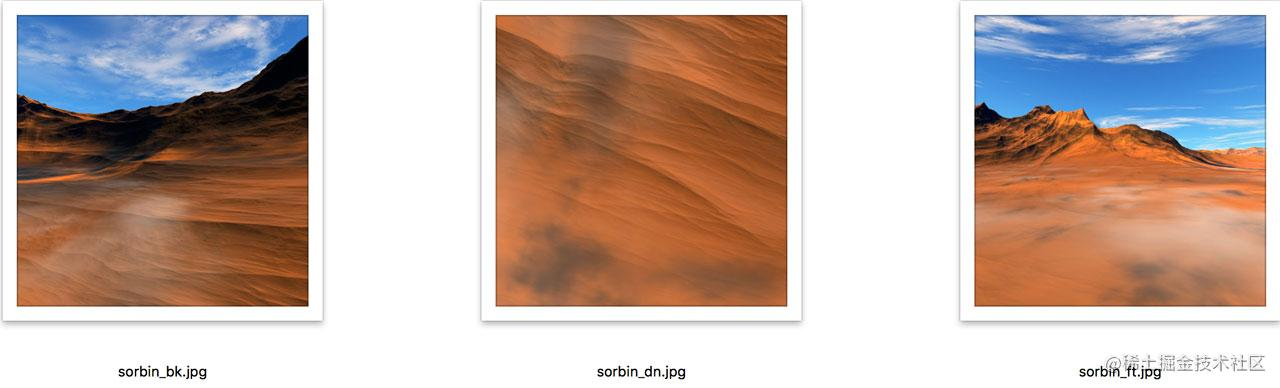# WebGL学习之纹理盒

``````gl.TEXTURE_CUBE_MAP_POSITIVE_X//右
gl.TEXTURE_CUBE_MAP_NEGATIVE_X//左
gl.TEXTURE_CUBE_MAP_POSITIVE_Y//上
gl.TEXTURE_CUBE_MAP_NEGATIVE_Y//下
gl.TEXTURE_CUBE_MAP_POSITIVE_Z//后
gl.TEXTURE_CUBE_MAP_NEGATIVE_Z//前

## 环境贴图

### 纹理``````// 创建纹理。
var texture = gl.createTexture();
gl.bindTexture(gl.TEXTURE_CUBE_MAP, texture);

const faceInfos = [
{
target: gl.TEXTURE_CUBE_MAP_POSITIVE_X,
url: '/img/sorbin_rt.jpg',
},
{
target: gl.TEXTURE_CUBE_MAP_NEGATIVE_X,
url: '/img/sorbin_lf.jpg',
},
{
target: gl.TEXTURE_CUBE_MAP_POSITIVE_Y,
url: '/img/sorbin_up.jpg',
},
{
target: gl.TEXTURE_CUBE_MAP_NEGATIVE_Y,
url: '/img/sorbin_dn.jpg',
},
{
target: gl.TEXTURE_CUBE_MAP_POSITIVE_Z,
url: '/img/sorbin_bk.jpg',
},
{
target: gl.TEXTURE_CUBE_MAP_NEGATIVE_Z,
url: '/img/sorbin_ft.jpg',
},
];
faceInfos.forEach((faceInfo) => {
const {target, url} = faceInfo;
// 上传画布到立方体贴图的每个面
const level = 0;
const format = gl.RGBA;
const width = 512;
const height = 512;
const type = gl.UNSIGNED_BYTE;
// 设置每个面，使其立即可渲染
gl.texImage2D(target, level, format, width, height, 0, format, type, null);

// 异步加载图片
const image = new Image();
image.src = url;
// 图片加载完成将其拷贝到纹理
gl.bindTexture(gl.TEXTURE_CUBE_MAP, texture);
gl.texImage2D(target, level, internalFormat, format, type, image);
gl.generateMipmap(gl.TEXTURE_CUBE_MAP);
};
});
gl.generateMipmap(gl.TEXTURE_CUBE_MAP);
gl.texParameteri(gl.TEXTURE_CUBE_MAP, gl.TEXTURE_MIN_FILTER, gl.LINEAR_MIPMAP_LINEAR);

### 法向量

3D立方体使用纹理盒有一个巨大的好处就是不需要额外指定纹理坐标。只要盒子是被放置在世界坐标系的原点，盒子本身的坐标就可以作为纹理坐标使用，因为在3D世界中位置本身就是一个向量，表示一个方向，我们要的就是这个方向。

``````attribute vec4 a_position;
uniform mat4 u_vpMatrix;
varying vec3 v_normal;

void main() {
gl_Position = u_vpMatrix * a_position;
//因为位置是以几何中心为原点的,可以用顶点坐标作为法向量
v_normal = normalize(a_position.xyz);
}

``````precision mediump float; // 从顶点着色器传入。
varying vec3 v_normal; // 纹理。
uniform samplerCube u_texture;

void main() {
gl_FragColor = textureCube(u_texture, normalize(v_normal));
}

### 实现

``````const viewPosition = new Vector3([0,0,1]);//相机位置
const lookAt = [0, 0, 0];//原点

//相机绕y轴旋转
cameraMatrix.rotate(0.2,0,1,0);
viewPoint = cameraMatrix.multiplyVector3(viewPosition);
vpMatrix.setPerspective( 30, canvas.width / canvas.height, 0.1, 5 );
vpMatrix.lookAt(...viewPoint.elements, ...lookAt, 0, 1, 0);

//重置位移
vpMatrix.elements = 0;
vpMatrix.elements = 0;
vpMatrix.elements = 0;

// 禁止写入深度缓存，造成背景在很远的假象

# 环境纹理映射

### 实现

``````attribute vec4 a_position;
attribute vec4 a_normal;
uniform mat4 u_vpMatrix;
uniform mat4 u_modelMatrix;
varying vec3 v_position;
varying vec3 v_normal;

void main() {
v_position = (u_modelMatrix * a_position).xyz;
v_normal = vec3(u_modelMatrix * a_normal);
gl_Position = u_vpMatrix * u_modelMatrix * a_position;
}

``````precision highp float;
varying vec3 v_position;
varying vec3 v_normal;
uniform samplerCube u_texture;
uniform vec3 u_viewPosition;

void main() {
vec3 normal = normalize(v_normal);
vec3 eyeToSurfaceDir = normalize(v_position - u_viewPosition);
vec3 direction = reflect(eyeToSurfaceDir,normal);
gl_FragColor = textureCube(u_texture, direction);
}

``````function draw(){
gl.clear(gl.COLOR_BUFFER_BIT | gl.DEPTH_BUFFER_BIT);

//天空盒
gl.useProgram(program.program);
//绘制天空盒
//...

//立方体
gl.useProgram(cProgram.program);
//绘制立方体
//...

requestAnimationFrame(draw);
}